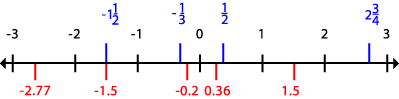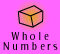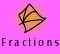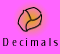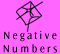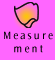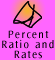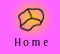| Teaching negative number arithmetic | Models for negative number arithmetic |
| The negative sign and subtraction | Building the number line |

Teaching negative number arithmetic

Children's use of negative numbers starts when they first count backwards and continue past zero. From 10,9,8,7,6,5,4,3,2,1,blast off, children as young as 6 or 7 will often find out about zero and below.

Examples such as a lift going up and down from a basement, temperature variations and submarines rising to and going below the surface of the water, and years BC and AD, are useful real world illustrations of how negative numbers can be used. Some real world examples, such as temperature, actually use negative numbers in the everyday representation. The other examples draw on the same idea but use a different way to represent it (e.g. having debts in red writing and credits in black, using B3 for the basement 3 below ground, using BC and AD).

By late primary school children should have developed some counting ability (but not calculation ability) with negative numbers. They may intuitively think about them on a vertical number line. A vertical number line showing negative numbers going below zero (such as height above and below sea level) relates more directly to the real world sense than a horizontal number line, so can be used first. However, it takes up a lot of space in written texts, so we have not used it here. Children should be able to compare numbers in real world situations, such as understanding that ­1 °C is warmer than ­3 °C.

Learning about negative number arithmetic is more difficult. So, for example, the calculation of an expression such as ­3 + (-4 + 7) = 0 builds on the intuitive understanding and counting work above, but is taught in secondary school. Children in junior secondary may start by playing grid games in all four quadrants. The fact that -1 x -1 = +1 is a deep puzzle to many people, which depends on generalising number properties and cannot be readily modelled (but is explained in the section on multiplication here).

In secondary school children are often taught 'rules' to help them remember how to do negative number arithmetic, such as 'two negatives make a positive'. However it is important to note that these 'rules' can lead to misunderstandings, such as -10 - 6 = 16. There are two negative signs in this question, but the result is not positive!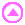Models for negative number arithmetic

As noted above, learning about negative numbers is not conceptually difficult and there are many simple models that illustrate basic ordering concepts. Learning to calculate, however, is conceptually hard. The meanings of operations (addition, subtraction, multiplication and division) can be developed using concrete models (see below). There are several models but there is still no model that adequately explains all the operations involving negative numbers. The understanding needs to come from the properties of numbers not from concrete models, particularly for multiplication and division by negative numbers. The models are described below and summarised in the table.

Annihilation model

We have used the annihilation model on the CD-ROM to model addition, subtraction and some examples of multiplication and division of directed numbers. In this model, a positive number is represented by a set of chips marked as +1 (so the positive number +3 is represented by three chips) and a negative number is represented by a set of chips marked as -1 (so the negative number -4 is represented by four chips).

 +3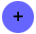-4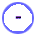+1 + (-1) = 0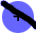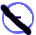A positive and a negative chip together annihilate each other, modelling the relationship +1 + (-1) = 0. The model is called the annihilation model for this reason. Using the annihilation property, a number can be represented in many different ways. For example, +3 can be represented by 10 positive chips and 7 negative chips. We chose this model because it illustrates the operations of addition and subtraction very clearly.

Addition of two numbers is modelled by combining two sets of chips (and annihilating pairs where possible).

So (+3) + (-4) is represented by the combined set of chips +++ ---- which, after the annihilation step is (-1).

Subtraction is modelled by take away, a common and easy interpretation of subtraction. How this is done is demonstrated elsewhere in this package.

Multiplication by a positive whole number is modelled by repeated addition. Selected divisions can be modelled by sharing into groups (partition) or by quotition.

However this model does not adequately explain multiplication of a negative number by a negative number.

Note: Some text books and teachers adapt the model to show this property, but this is not logically correct. Here is an example for you NOT to follow.

"In order to use the model to show negative 4 lots of negative 3, -4 x ­3 is interpreted as take away 4 times negative 3.

"But, in order to take away 4 times ­3 I need to have 4 times ­3 to start with.

"So I can start with 4 times 3 positive chips and 4 times 3 negative chips (because this equates to zero) and then I can take away 4 times ­3. After doing so I am left with 4 times 3 positive chips."

In this example, the negative sign in front of a number is used in two different ways: once as the unary sign which indicates a negative number and once as the binary sign which indicates subtraction. This failure of the model cannot be overcome ­ the multiplication of two negative numbers must be justified in another way, as was done elsewhere on the CD-ROM.

Pebbles in a bag model

In this model, numbers are represented by CHANGES to the number of pebbles in a bag. Start with a big bag containing a large unknown number of pebbles. Positive numbers are represented by adding pebbles to the bag and negative numbers by removing pebbles from the bag.

The number + 3, for example, is represented by ADDING 3 pebbles to the bag.

The number -4, for example, is represented by TAKING 4 pebbles from the bag.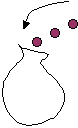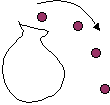+ 3 is represented by ADDING 3 pebbles to the bag. -4 is represented by TAKING 4 pebbles from the bag

Children can take part by having their own bags to work with. Adding numbers is carrying out these changes successively.

So +3 + (-4) is equal to ­1 because adding 3 pebbles to the bag, then taking out four (+3 + (-4)) gives the same results as taking one out of the bag (-1).

Multiplication by a positive whole number is repeated addition (so 6 times -4 is carrying out the -4 change 6 times which results in removing 24 pebbles from the bag). Division is treated as the inverse operation.

Number line model

Negative and positive numbers can be modelled using a number line. However, when using the number line to illustrate operations, it is important to clarify whether numbers are represented by positions on the line or movements along the line or BOTH. This is a source of confusion that needs careful attention, especially so that the teacher has their ideas well sorted out. Children can physically take part by carrying out the operations of moving along the line.

In the simplest model, numbers are modelled by positions on the number line (so + 3 is the position 3 units to the right of zero on the line and ­4 is the position 4 units to the left of zero). They can also be modelled by changes of position, positive to the right and negative to the left.

(+6) +(+7) = +13

We start at position +6, then move 7 units right, reaching position +13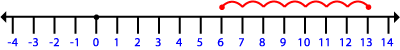+6 +(-7) = -1

We start at position +6, then move 7 units left, reaching position ­1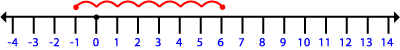Multiplication is repeated addition i.e. repeated changes:

5 x (+3) = +3 +(+3) +(+3) +(+3) +(+3) = +15 we start at position +3, then move 3 units right four times, reaching position +15 (Alternatively, start at position 0 and move 5 times by 3 units)

5 x (-4) = -4 +(-4) +(-4) +(-4) +(-4) = -20 we start at position -4, then move 4 units left four times, reaching position ­20

Subtraction and division are treated as inverse operations

Soldier model

Imagine a soldier standing on a point marked X on a parade ground and initially facing north. Numbers are modelled by the soldier's movements as he or she moves forwards and backwards. (He never goes sideways).

So + 3 means move 3 steps forward (in the direction the soldier is facing), ­4 means move 4 steps backward (still facing the same way).

The operation + instructs the soldier to face north, which may involve an about turn. The operation ­ instructs the soldier to face south, turning if necessary.

Notice that positive means forward, whereas addition means face north. Negative means backwards, whereas subtraction means face south.

(+6) +(+7) = +13 Starting at X, our soldier moves 6 steps forward, then facing north he moves 7 steps forward. This double movement is the same as the single movement of +13 (move 13 steps north) so (+6) + (+7) = +13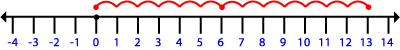This same diagram represents the problem (+6) -(-7) = +13 although the movements are different. Starting at X, our soldier moves 6 steps forward, then facing south he moves 7 steps backwards, ending up 13 steps north of X again.

(+6) +(-7) = (-1) Starting at X, the soldier moves 6 steps forward, then facing north still, he moves backward 7 steps. This is the same as a movement of one step backwards from X, so (+6) +(-7) = (-1).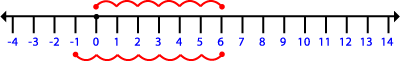This same diagram also represents the problem (+6) - (+7) = (-1) although the movements are different. Starting at X, the soldier moves 6 steps forward, then facing south, he moves forward 7 steps, ending up again at one step south of X.

Multiplication and division

Multiplication by a positive whole number is repeated addition i.e. repeated changes:

5 x (+3) = (+3) +(+3) +(+3) +(+3) +(+3) = +15 Starting at X, he moves 3 steps forward five times (without turning around), which is the same as one movement forward of 15 steps from X.

5 x (-4) = -4 +(-4) +(-4) +(-4) +(-4) = -20 Starting at X, he moves 4 steps backwards five times (without turning around), which is the same as one movement backward of 20 steps.

Division is treated as the inverse operation.

Summary of models

 MODEL: Annihilation Pebbles in a bag Number line Soldiers Representation of number Sets of chips Change in the number of pebbles in the bag Either a position on a line or a movement along the line Movement forwards or backwards along the line Representation of addition Combining two sets of chips following one change by another Start at a position and move to another One movement followed by another, facing north Representation of subtraction Taking away chips from a given set Inverse of addition Movement to go between positions One movement followed by another, facing the south Representation of multiplication by a positive number Repeated addition Repeated addition Repeated addition Repeated addition Representation of multiplication of a positive number by a negative number Commutative law Commutative law Commutative law Commutative law Representation of multiplication of a negative number by a negative number Pattern continuation Pattern continuation Pattern continuation Pattern continuation Representation of division by a positive number sharing into groups (partition division) Inverse of multiplication Inverse of multiplication Inverse of multiplication Representation of division of a positive number by a negative number how many groups (quotition division), Inverse of multiplication Inverse of multiplication Inverse of multiplication Inverse of multiplication Representation of division of a negative number by a negative number Inverse of multiplication Inverse of multiplication Inverse of multiplication Inverse of multiplicationThe negative sign and subtraction

As we have mentioned in the CD-ROM content it is important to make the distinction between a negative sign (the unary sign, which applies to one number) and subtraction (the binary sign which connects two numbers). As confident users of negative number arithmetic this distinction between the two signs is not something we even think about, but it is important to be aware of it as teachers and be consistent in our terminology and use of the signs so that students develop a clear understanding of what the symbols mean in each context.Building the number line

It is very important that children gradually build up the idea of one number line that holds all real numbers, otherwise children may have the misconception that decimals fit on one number line, whole numbers on another and fractions on another. They also need to become comfortable with negative integers, negative decimals and negative fractions and their position below zero. Children need to see that all these numbers can be accommodated on the one number line.